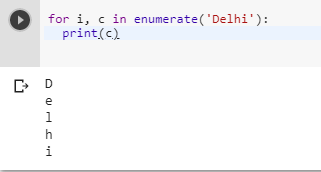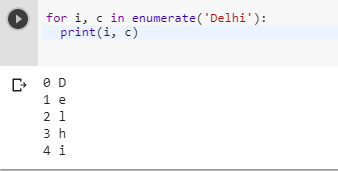# Iterating each character in a string using Python

0 votes
1 view
in Python

In C++, I can iterate over an std::string like this:

std::string str = "Hello World!";

for (int i = 0; i < str.length(); ++i)

{

std::cout << str[i] << std::endl;

}

How do I iterate over a string in Python?

## 1 Answer

0 votes
by (107k points)

There are many ways to iterate over a string in Python:-

The first thing you can use a for loop to iterate over a given string like as follows:-

for c in "Delhi":

print(c)Another way is to use enumerate()so If you need access to the index as you iterate through the string, by using enumerate() below is the code that explains the use of enumerate():-

for i, c in enumerate('Delhi'):

print(i, c)0 votes
1 answer
0 votes
1 answer
0 votes
1 answer
0 votes
1 answer
0 votes
1 answer